Up: Time Correlation Functions. Random Previous: Spectral Representation

Subsections

# Random Force and Its Applications

## Stochastic Equation

We wrote kinetic equation for the average x as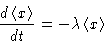What happens if we want to measure x for one system in the ensemble? Then we write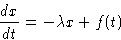(6)
with random variable x. The function f(t) is random force.

## Properties of Random Force

We can speak only about average properties of f(t). We will calculate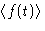and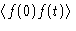. We will average over equilibrium ensemble.

Averaging (6), we obtain: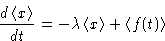or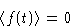(7)
Equation (6) can be solved. The solution is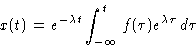(8)
Correlation function: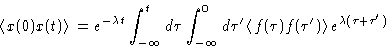But we know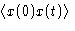! Result: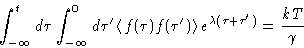This could be rewritten as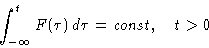This is possible only if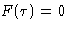for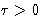But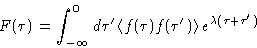and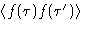depends only on. We obtain: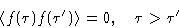From symmetrySinceis not zero, it is a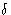-function: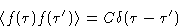Then we have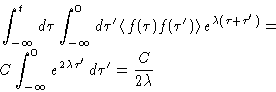and since this is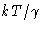,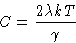We obtained:(9)
or, after Fourier transform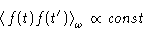(10)

## White Noise and Colored Noise

Sometimes the function f(t) is called random noise. Equation (9) shows that random noise is-correlated. In other words, all harmonics are present in its Fourier transform with equal weights (equation (10)). Such noise is called white noise (remember rainbow?).

Colored noise: not all harmonics are equal: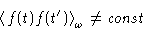and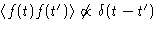.

How can it be so? What's wrong in our derivation?

We started from the equation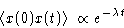(11)
This is a consequence of the fact that x is the slowest mode in the system and we averaged out everything else! If this is not true, equation (11) does not work, and noise is no longer white!

Another interpretation: the-function in equation (9) is the-function only approximately. In fact it is a sharp peak with the width about the characteristic time of the molecular processes.Up: Time Correlation Functions. Random Previous: Spectral Representation

© 1997 Boris Veytsman and Michael Kotelyanskii
Tue Oct 28 22:16:24 EST 1997